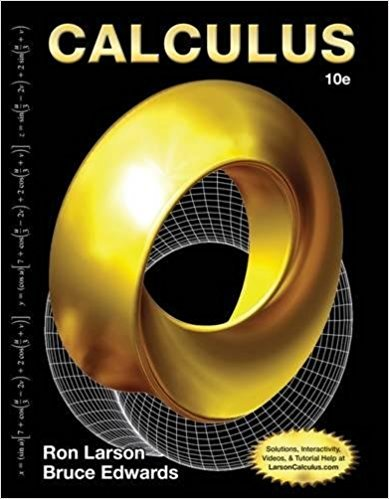×
×

# Solutions for Chapter 10: Calculus 10th Edition## Full solutions for Calculus | 10th Edition

ISBN: 9781285057095Solutions for Chapter 10

Solutions for Chapter 10
4 5 0 251 Reviews
10
0
##### ISBN: 9781285057095

Since 17 problems in chapter 10 have been answered, more than 3030 students have viewed full step-by-step solutions from this chapter. Calculus was written by and is associated to the ISBN: 9781285057095. Chapter 10 includes 17 full step-by-step solutions. This textbook survival guide was created for the textbook: Calculus, edition: 10. This expansive textbook survival guide covers the following chapters and their solutions.

Key Calculus Terms and definitions covered in this textbook

If u = v and w = z , then u + w = v + z

• Circle graph

A circular graphical display of categorical data

• Common difference

See Arithmetic sequence.

• Elements of a matrix

See Matrix element.

• Elimination method

A method of solving a system of linear equations

• Equilibrium price

See Equilibrium point.

• Extraneous solution

Any solution of the resulting equation that is not a solution of the original equation.

• First quartile

See Quartile.

• Fundamental

Theorem of Algebra A polynomial function of degree has n complex zeros (counting multiplicity).

• Horizontal component

See Component form of a vector.

• Identity matrix

A square matrix with 1’s in the main diagonal and 0’s elsewhere, p. 534.

• Infinite limit

A special case of a limit that does not exist.

• Limit at infinity

limx: qƒ1x2 = L means that ƒ1x2 gets arbitrarily close to L as x gets arbitrarily large; lim x:- q ƒ1x2 means that gets arbitrarily close to L as gets arbitrarily large

• Logarithmic regression

See Natural logarithmic regression

• Natural logarithmic regression

A procedure for fitting a logarithmic curve to a set of data.

• Origin

The number zero on a number line, or the point where the x- and y-axes cross in the Cartesian coordinate system, or the point where the x-, y-, and z-axes cross in Cartesian three-dimensional space

• Polar equation

An equation in r and ?.

• Standard form of a polar equation of a conic

r = ke 1 e cos ? or r = ke 1 e sin ? ,

• Ymax

The y-value of the top of the viewing window.

• Ymin

The y-value of the bottom of the viewing window.

×